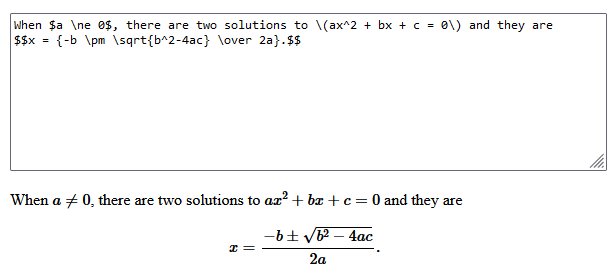# Using Latex with Xojo for displaying Maths equations

Hi all, I would like to be able to use Xojo to display Maths equations written in Latex, but so far have not been able to determine if Xojo can do this and if so how. Any ideas would be appreciated. I am a Maths teacher and want to show students how the order that operations are applied to a variable will affect the final expression. For example x mult by 2 and then add 3 gives a different expression to x add 3 then mult by 2. (Obvious to us but not to all students). Many thanks in anticipation.

Maybe give a link to the Latex program/website as searching it gives interesting results lol

Search for [LaTex editor]. For example

LaTeX - A document preparation system (latex-project.org)

There are many providers for LaTex compliant software.

omg! Is this software still being used? How about using Mathematica instead?

I guess the easiest way would be to use Xojo as a frontend for KaTeX. The following link could give you an idea on how to accomplish this.

And by the way: LaTeX is still the gold standard when it comes to rendering mathematical expressions or writing scientific papers1 Like

Hi people, I am not sure I explained myself clearly enough. I am a Secondary Maths teacher. One of the difficulties our less able students have is understanding how an algebraic expression has been built up. This is important when evaluating an expression, when solving equations and just generally understanding algebra. it is also important in calculus when differentiating using the ‘function of a function’ or ‘chain rule’.

I had thought it might be good to have a little app that can be used to demonstrate the principles. It would be in the form of a ‘function machine’ when students would choose an operation (like +4 or x5 or square etc) and the app would display the resulting expression.

To achieve this I would need Xojo to be able to display correct mathematical expressions or equations. I would like to do this without writing my own code (as I did back in the days of the Apple ][ ). For example in algebra we write division using fractions. Calculates offsets etc is not a simple task but Latex does a good job with this as I thing does Math ML

Can I achieve this in any simple way with Xojo?

I hated Latex with the odd spelling over 25 years ago when I needed to do my thesis.

You can try to load Math ML into a html viewer.

I have a Macintosh only solution.
I wrote this code some time ago. It converts a LaTeX math expression into an image of the formatted expression. It uses a shell command to call pdflatex and dvipng which are part of the MacTex/TexLive distributions.

Public Function Latex2pic(mTxt As String, res As Integer) as Picture
'Convert a LaTeX format math formula string to a picture
'mTxt is the LaTeX text
'res is the required picture resolution in dpi
'Uses a shell to call pdflatex and dvipng commands
'which are part of the MacTex/TexLive distributions

'cmdPath is the path to the directory of the Tex commands.
'Depending on which Tex distribution is used this may need to be changed.
dim cmdPath As String = "/usr/local/texlive/2018/bin/x86_64-darwin/"

dim f As FolderItem = SpecialFolder.ApplicationData.Child("MyAppStuff")
if not f.Exists then
'Create Application Support/MyAppStuff folder.
f.CreateAsFolder
end if
dim sPath As String = f.NativePath

Dim My_Shell As New Shell
My_Shell.TimeOut=-1
Dim theShellCode As String = "cd """+sPath+""""+EndOfLine
theShellCode = theShellCode + cmdPath+"pdflatex -output-format=dvi <<<'"
theShellCode = theShellCode + "\documentclass[12pt]{slides}"+EndOfLine
theShellCode = theShellCode + "\usepackage{geometry}"+EndOfLine
theShellCode = theShellCode + "\usepackage{lingmacros}"+EndOfLine
theShellCode = theShellCode + "\usepackage{tree-dvips}"+EndOfLine
theShellCode = theShellCode + "\usepackage[usenames]{color} %used for font color"+EndOfLine
theShellCode = theShellCode + "\usepackage{amssymb} %maths"+EndOfLine
theShellCode = theShellCode + "\usepackage{amsmath} %maths"+EndOfLine
theShellCode = theShellCode + "\usepackage[utf8]{inputenc} %useful to type directly diacritic characters"+EndOfLine
theShellCode = theShellCode + "\geometry{papersize={8.5in,1.5in}}"+EndOfLine
theShellCode = theShellCode + "\begin{document}"+EndOfLine
'Insert latex math code here:
theShellCode = theShellCode + mTxt+EndOfLine
'End of math code
theShellCode = theShellCode + "\end{document}'"+EndOfLine
theShellCode = theShellCode + cmdPath+"dvipng -bg Transparent -D "+str(res)+" texput.dvi"+EndOfLine
My_Shell.Execute theShellCode
'If everything went according to plan, there should be a png file in the app's app support folder.
dim picFile As FolderItem = f.Child("texput1.png") 'default png file name
If picFile <> Nil Then
Dim pic As Picture
pic = Picture.Open(picFile)
'Optionally, place the picture directly in the window with either of the following uncommented:
if pic.Width>ImageWell1.Width-30 or pic.Height>ImageWell1.Height-10 then
ImageWell1.Image = ScaleImage(pic,ImageWell1.Width-100,ImageWell1.Height-10)
Else
ImageWell1.Image = pic
End If

if pic.Width>Canvas1.Width-30 or pic.Height>Canvas1.Height-10 then
Canvas1.Backdrop = ScaleImage(pic,Canvas1.Width-100,Canvas1.Height-10)
Else
Canvas1.Backdrop = pic
End If

Return pic
Else
'Error - Couldn't open picFile
return nil
End If
End Function
2 Likes

Thank you Robert. Will certainly try this out and see how it goes. Given that I’m teaching it may be a few days before I can try it out. But again Thanks.

Hi Karl,

Here is something I quickly made using a WebHTMLViewer. You could also use this code in any of the project types but you’d have to do that manually. The webpage code was pulled from the demo for MathJax | Beautiful math in all browsers.Just the module

Test Web Project

You can do a lot in Xojo with the provided tools

2 Likes